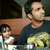0

# Plots out of view when plotting using reversed y axes

Deepak Gopalakrishnan 3 years ago updated 3 years ago

I modified the AxisExample - Reversed like below

```<code>
public static PlotModel Reversed()
{
var plotModel1 = new PlotModel();
{
Position = AxisPosition.Left,
StartPosition = 55,
EndPosition = 0,
MajorStep = 10,
Key = "Y1"
});
{
Position = AxisPosition.Right,
StartPosition = 55,
EndPosition = 0,
MajorStep = 10,
Key = "Y2"
});
var series1 = new LineSeries();
series1.Color = OxyColors.Black;
series1.YAxisKey = "Y2";
var series = new LineSeries();
series.Color = OxyColors.Black;
series.YAxisKey = "Y1";
{
Position = AxisPosition.Bottom,
Minimum = 0,
Maximum = 35,
MajorStep = 10,
MinorStep = 5,
});
return plotModel1;
}
</code>```

The resulting plot:

After zooming and panning (expected results):

What is going wrong in my code? Not able to figure out.

Thanksif I set

```<code>
EndPosition = 0,
StartPosition = 1,
</code>```

It seems to work.

Not sure why

```StartPosition = 55,
EndPosition = 0,```

Didnt work.